• 1、 分段函数可导判断 2、含绝对值函数 3、带极限的函数
1、 分段函数可导性判断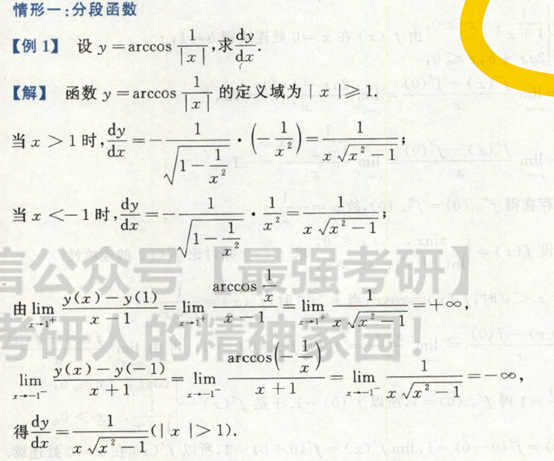2、含绝对值函数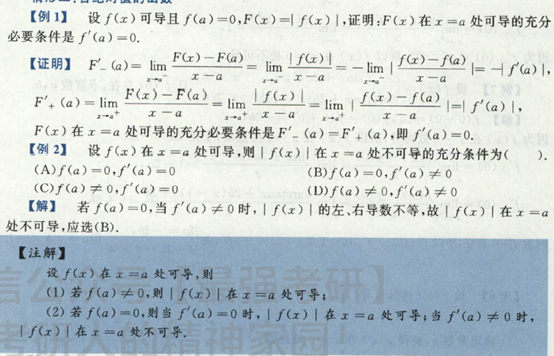3、带极限的函数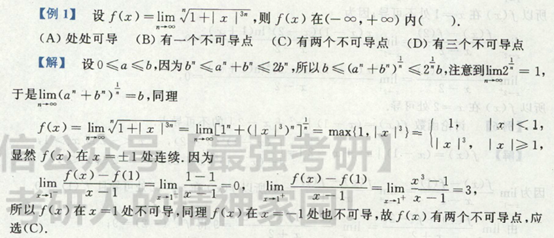展开全文• 虽然求可导点方法很多，但是我觉得这个方法是最简单最快捷的。 原创视频：
虽然求可导点方法很多，但是我觉得这个方法是最简单最快捷的。 原创视频：<点这里>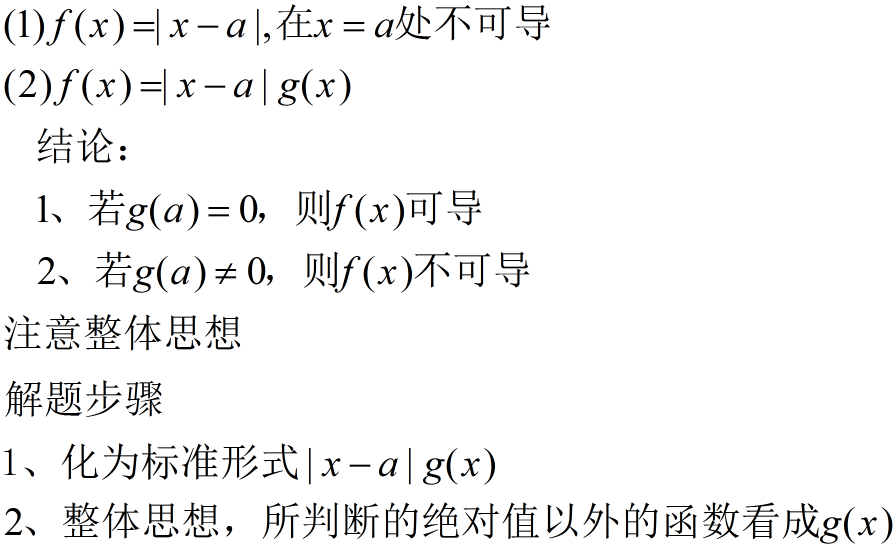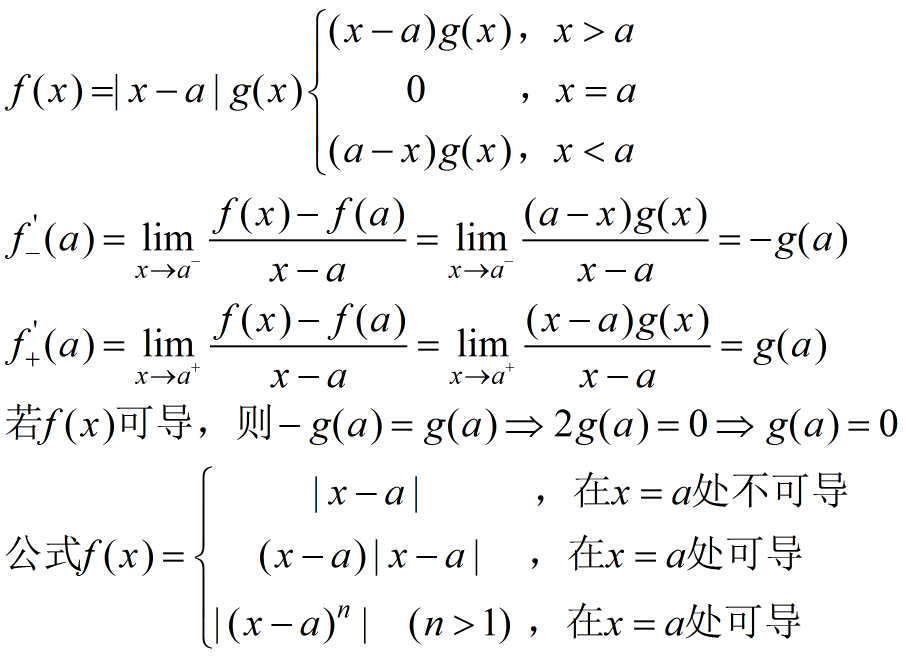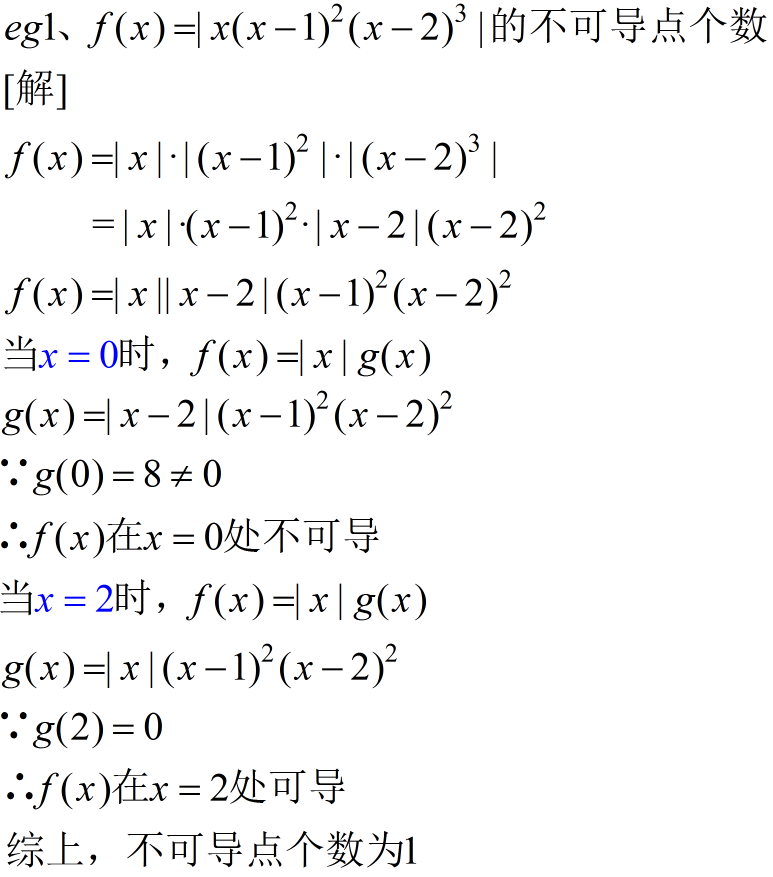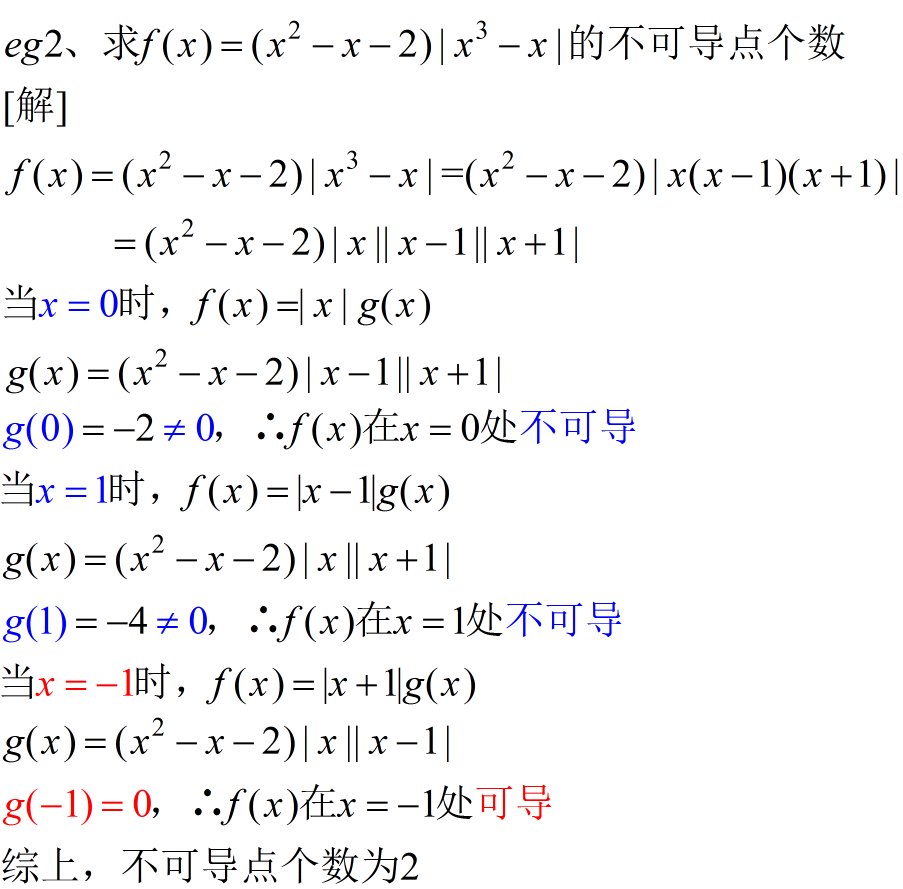展开全文高等数学 期末考试
• 西城学探诊高中数学3.3.2利用导数判断函数的极值最值学案无答案新人教B版选修1_1
• 西城学探诊高中数学3.3.1利用导数判断函数的单调性学案无答案新人教B版选修1_1
• ## 复合函数的可导性

千次阅读 2020-09-04 09:00:33
limh→01☸f(☣)存在 \left.... 并且内部函数与分母h的函数同阶（右侧不能为零，为零的话就可能不可导了，无穷时也不行，注意是充要条件） D项构造在零点分段的函数，从而导出可导与零点无关。 答案：B


l

i

m

h

→

0

1

☸

f

(

☣

)

存

在

\left. \begin{array} { l } { l i m } \\ { h \rightarrow 0 } \end{array} \right. \frac{1}{☸} f(☣)存在

=

l

i

m

h

→

0

1

☣

f

(

☣

)

∗

☣

☸

存

在

= \left. \begin{array} { l } { l i m } \\ { h \rightarrow 0 } \end{array} \right. \frac{1}{☣} f(☣)*\frac{☣}{☸}存在

看内部函数☣的0的两个方向趋向（并且在求导点的邻域不能有零值），
并且内部函数与分母h的函数☸同阶（右侧相除的极限不能为零，为零的话就可能不可导了，无穷时也不行，注意是充要条件）
例题： f(0)=0,则f(x)在x=0处可导的重要条件为： a)

l

i

m

h

→

0

1

h

2

f

(

1

−

c

o

s

h

)

存

在

\left. \begin{array} { l } { l i m } \\ { h \rightarrow 0 } \end{array} \right. \frac{1}{h^2} f(1-cosh)存在

b)

l

i

m

h

→

0

1

h

f

(

1

−

e

h

)

存

在

\left. \begin{array} { l } { l i m } \\ { h \rightarrow 0 } \end{array} \right. \frac{1}{h} f(1-e^h)存在

c)

l

i

m

h

→

0

1

h

2

f

(

h

−

s

i

n

(

h

)

)

存

在

\left. \begin{array} { l } { l i m } \\ { h \rightarrow 0 } \end{array} \right. \frac{1}{h^2} f(h-sin(h))存在

d)

l

i

m

h

→

0

1

h

(

f

(

2

h

)

−

f

(

h

)

)

存

在

\left. \begin{array} { l } { l i m } \\ { h \rightarrow 0 } \end{array} \right. \frac{1}{h} (f(2h)-f(h))存在

看内部函数的0的两个方向趋向（并且在求导点的邻域不能有零值）， 并且内部函数与分母h的函数同阶（右侧不能为零，为零的话就可能不可导了，无穷时也不行，注意是充要条件）
D项可构造在零点分段的函数，从而导出可导与零点无关。
A 1-COSh只能趋于零正
答案：B
展开全文• 最近复习到高数的一元函数微分部分 ，可导和可微是两个特别重要也特别容易混淆的概念。所以简单记录一下，便于自己理解，仅供参考。 导数 从物理角度来说（牛顿是从物理学的角度发明出的微积分）某点的导数就是一个...
最近复习到高数的一元函数微分部分 ，可导和可微是两个特别重要也特别容易混淆的概念。所以简单记录一下，便于自己理解，仅供参考。
导数
从物理角度来说（牛顿是从物理学的角度发明出的微积分）某点的导数就是一个该点的瞬时变化率的问题。 几何意义上来说（莱布尼茨从数学角度发明出的微积分），某点的导数是曲线在该点处的切线的斜率。 从定义来看，导数在本质上是一个极限问题。f(x)在

x

0

x_0

处的导数

f

(

x

)

ˋ

\grave{f(x)}

为：

f

(

x

)

ˋ

=

lim

⁡

Δ

x

→

0

f

(

x

0

+

Δ

x

)

−

f

(

x

0

)

Δ

x

\grave{f(x)}=\lim_{\Delta x\to \ 0} \frac{f(x_0+\Delta x)-f(x_0)}{\Delta x}

若该极限存在， 就说明函数在该点可导。当然我们知道，函数极限也可能不存在，所以该点也就不可导了。
微分：
从数形结合的方式理解：设一正方形边长为x，当边长增加

Δ

x

\Delta x

,面积增加

Δ

S

=

(

x

+

Δ

x

)

2

−

x

2

=

2

x

Δ

x

−

Δ

x

2

\Delta S=(x+\Delta x)^2-x^2=2x\Delta x-\Delta x^2

当

Δ

x

→

0

{\Delta x\to \ 0}

时，

(

Δ

x

)

2

(\Delta x)^2

对于

Δ

x

\Delta x

来说是高阶无穷小,记为

o

(

Δ

x

)

o(\Delta x)

，可以看作误差， 忽略不计。

2

x

Δ

x

2x\Delta x

才是增量的主要部分，我们叫做主部。
推广至一般函数，对于函数

f

(

x

)

f(x)

在

x

0

x_0

的某领域内有定义，那么函数增量记为：

Δ

y

=

f

(

x

+

Δ

x

)

−

f

(

x

)

\Delta y=f(x+\Delta x)-f(x)

若存在与

Δ

x

\Delta x

无关的常数

A

A

使得

Δ

y

=

A

Δ

x

+

o

(

Δ

x

)

\Delta y=A\Delta x+o(\Delta x)

,那么称

f

(

x

)

f(x)

在

x

0

x_0

处可微，微分为

A

Δ

x

A\Delta x

，即增量的线性主部。记作

d

y

∣

x

=

x

0

=

A

Δ

x

dy\lvert _{x=x_0}=A\Delta x

。 （其实这里的常数

A

A

就是

f

(

x

)

f(x)

在该点处的导数） 又因为

Δ

x

=

d

x

\Delta x=dx

（这里要理解一下为什么

Δ

x

=

d

x

\Delta x=dx

其实严格意义上

Δ

x

=

d

x

\Delta x=dx

因为一个是增量，一个是微分。 根据上面微分的定义 我们可以知道：

d

x

=

A

Δ

x

+

o

(

x

)

dx=A \Delta x+o(x)

所以他们之间有一个无穷小的误差。我们忽略不计）

d

y

∣

x

=

x

0

=

A

Δ

x

=

y

(

x

)

ˋ

Δ

x

=

y

(

x

)

ˋ

d

x

dy\lvert _{x=x_0}=A\Delta x=\grave{y(x)} \Delta x=\grave{y(x)} dx

d

y

d

x

∣

x

=

x

0

=

y

(

x

)

ˋ

d

x

d

x

=

y

(

x

)

ˋ

\frac{dy}{dx}\lvert _{x=x_0}=\frac{\grave{y(x)} dx}{dx}=\grave{y(x)}

通过上面的两个公式我们可以推出一元函数 可微必可导 两者互为充要条件。所以判断

f

(

x

)

f(x)

在

x

0

x_0

处是否可微就可以转换成是否可导的问题。 关于可微的含义：我们用线性增量

A

Δ

x

A \Delta x

代替复杂增量

Δ

y

\Delta y

,误差为

Δ

y

−

A

Δ

x

=

o

(

x

)

\Delta y - A\Delta x=o(x)

无穷小，可忽略不计。
几何意义：若

f

(

x

)

f(x)

在

x

0

x_0

处是否可微，则在

（

x

0

,

y

0

)

（x_0,y_0)

处可以用切线段(线性增量

A

Δ

x

A\Delta x

）近似替代曲线段（实际复杂增量

Δ

y

\Delta y

）。这个和后面函数在某个定义域内的积分的几何意义联系起来了，也是用线段近似替代曲线。
展开全文• 函数可导，说明函数在该点有唯一变化率（变化趋势），对于没定义的点或者不连续的点，函数在该点一定不可导gradcheck PyTorch 深度学习 反向传播
• ## 判断函数凹凸性

千次阅读 2020-05-18 17:39:37
假设fff微，则函数fff是凸函数的充要条件是domfdom fdomf是凸集且对于任意x,y∈domfx, y∈domfx,y∈domf, f(y)⩾f(x)+∇f(x)T(y−x)f(y) \geqslant f(x)+\nabla f(x)^{T}(y-x)f(y)⩾f(x)+∇f(x)T(y−x)成立。...算法
• 通过几个例子指出判断函数在一点处是否可导要注意它的充分条件,剖析了错误判断产生的原因。
• 一、极值点 ...函数的拐点理解为导数的极值点，因此上述关于极值点的结论都“稍加改变”后用于判断拐点，下面是一些常用结论： 拐点的必要条件： 拐点的充分条件：且在左右两侧变号 利用三阶...
• 4，如果二元函数f(x,y)的偏数fx(x,y),fy(x,y)在点(x0,y0)连续，如果二元函数f(x,y)的偏数f_x(x,y),f_y(x,y)在点(x_0,y_0)连续，如果二元函数f(x,y)的偏数fx​(x,y),fy​(x,y)在点(x0​,y0​)连续， ...
• 前言 典例剖析 例1(给定$$f'(x)$$的图像，确定$$f(x)\...已知函数\(y=xf'(x)$$的图像如图所示(其中$$f'(x)$$是函数$$f(x)$$的导函数)，则下面四个图像中，$$y=f(x)$$的图像大致是【】 分析：由图可知， 当\(x<-1...
•算法 高等数学
• 对于一元函数f(x)f(x)，我们可以通过其二阶导数f′′(x)f″(x) 的符号来判断。如果函数的二阶导数总是非负，即f′′(x)≥0f″(x)≥0 ，则f(x)f(x)是凸函数 对于多元函数f(X)f(X)，我们可以通过其Hessian矩阵...
• 1.f(x)n阶导函数存在 <=======> f(n)(x)存在 指的是在某个区间内有定义 2.f(x)n阶可导根据题意可以有两种不同的解释： 　①.题目中说的是在某点即在x=x0处n阶可导，指的是f(n)(x0)存在。 　②.题目直接说...
• 首先定义凸集，如果x，y属于某个集合M...如果函数存在二阶并且为正，或者多元函数的Hessian矩阵半正定则均为凸函数。 「注意」：中国大陆数学界某些机构关于函数凹凸性定义和国外的定义是相反的。Convex Function...机器学习 算法 python 数学建模
• 以二元函数的二阶偏数为例，偏x导数有两个偏数、偏y导数有两个偏数。 定理：如果二元函数的两个二阶混合偏数连续，那么他们两个相等。 全微分 与一元函数类似，由于有两个变量，x或y的增量称为偏增量，单单...高等数学 全导数
• 本文介绍导数用于判断函数的单调性，凹凸性，极值和函数的最大值，最小值极小值
• 但是,函数在一点处解析与在一点处可导是不等价的概念. 即函数在一点处可导, 不一定在该点处解析. 但是,函数在一点处解析与在一点处可导是不等价的概念. 即函数在一点处可导, 不一定在该点处解析. 但是,函数在一点处...
• 在高等数学一元函数微分学中研究的关键问题之一是可导和可微，夹杂着函数连续，简短等知识点，这几个相关的概念混在一块总是难以理解，什么可导一定微，可导一定连续之类的。 这里把这几个概念就自己的理解做一下...
• 被积函数虽然积，但是不能表达变上限积分函数是否可导，除非被积函数连续。 但是只要被积函数可积，则变上限积分函数必定连续。 变上限积分的根子还是在函数的积分，因此我么只看不定积分的一般情况。 第一类...数学
• 关于用导数法判断函数的单调性问题，教材上所举例子是从数的角度求解函数的正负，从而判断原函数的单调性，所以学生就依葫芦画瓢，碰到这类问题都这样做，但是他会发现在高三中的大多数同类题目都不能求解，思路...
• 而偏连续则是更强的条件，即偏存在且连续可以推出多元函数连续，反之不。 下面来分析，首先大家需要了解这些定义都是人定义出来的，可以反映多元函数的部分特征。所以，只要掌握了这些定义的意义就可以看出其...
• 由图①可知，当一阶导函数在某点（即驻点）处为0，二阶导函数在该点处小于0时，原函数在该驻点处取极大值； 由图②可知，当一阶导函数在某点（即驻点）处为0，二阶导函数在该点处大于0时，原函数在该驻点处取极小值...驻点 二阶导数 最优化方法
• 2.编写代码进行测试，主要包括可导函数square和不可导函数floor 代码参考网站 https://research.googleblog.com/2017/10/eager-execution-imperative-define-by.html 代码示例： import numpy as np ...
• 二元函数凹凸性判断 二元函数凹凸性判断： 设f(x,y)f(x,y)f(x,y)在区域DDD上具有二阶连续偏数，且分别记为：A=fxx′′(x,y)，B=fxy′′(x,y)，C=fyy′′(x,y)A=f_{xx}^{''}(x,y)，B=f_{xy}^{''}(x,y)，C=f_{yy}^{'...机器学习 数据挖掘
• 函数极值点一定是驻点，驻点不一定是极值点，驻点为两个偏数存在且均为0的称为驻点 令A=，B=，​​​​​C= 若AC-B^2>0，则为极值点，且A>0为极小值 若AC-B^2<0，则不是极值点 若AC-B^2=0，则进一步......